# Toroidal Plasma Equilibrium

As before, let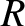,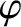,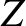be a set of right-handed cylindrical coordinates whose symmetry axis corresponds to that of the plasma equilibrium. On the other hand, let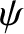,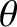,be a set of right-handed flux coordinates such that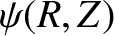labels the equilibrium magnetic flux-surfaces, andincreases by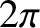for every poloidal circuit of a given flux-surface. We can assume that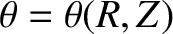without loss of generality. (Note thatis a generalization of the poloidal angle introduced in Section 2.7 that does not assume that the flux-surfaces have circular cross-sections.) As before, we shall set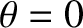on the outboard midplane. Note that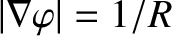. The Jacobean of our flux-coordinate system is defined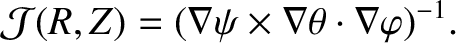(2.121)

Now, a general vector field,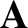, can be written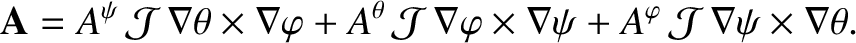(2.122)

Moreover ,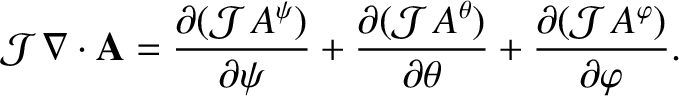(2.123)

The axisymmetric equilibrium magnetic field of a tokamak can be expressed in the following manifestly divergence-free manner: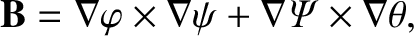(2.124)

where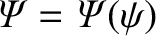. It follows that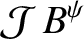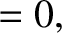(2.125)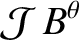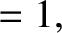(2.126)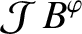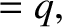(2.127)

where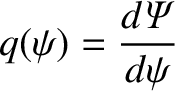(2.128)

is the safety-factor profile . Note that the previous expression reduces to expression (1.76) in the large aspect-ratio, circular magnetic flux-surface limit.

It is convenient to specialize to a coordinate system in which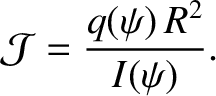(2.129)

It follows that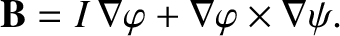(2.130)

The equilibrium electric field is written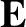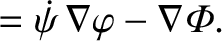(2.131)

Here,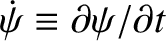, and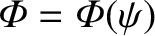. Note that the previous equation automatically satisfies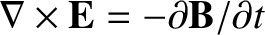.

Finally, we expect the plasma equilibrium to be characterized by number density, temperature, and pressure profiles that are flux-surface functions . In other words,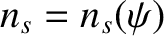,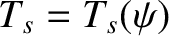, and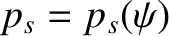. (See Section 2.25.)